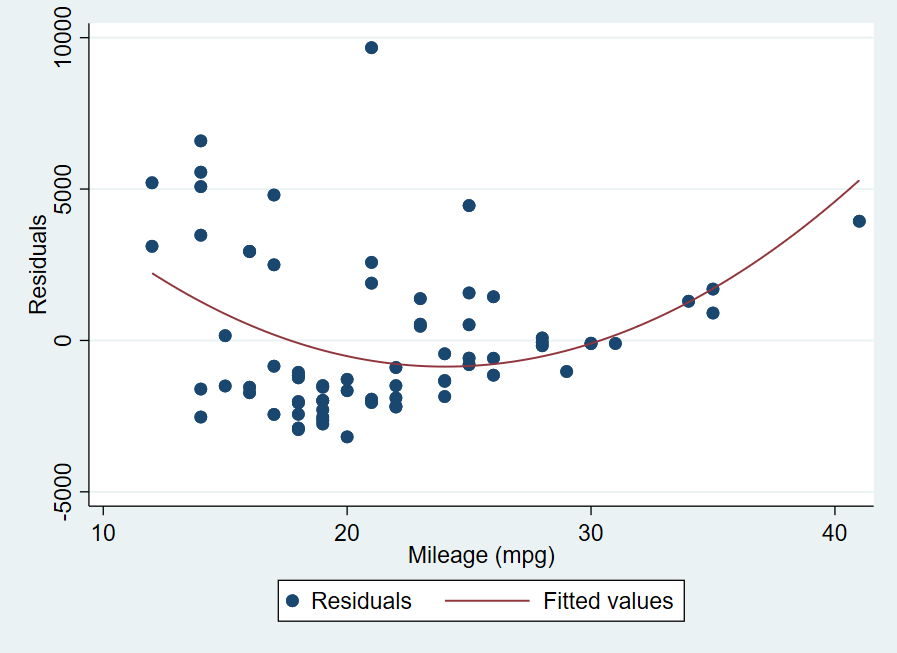Search

# The rvpplot Command - Linear Regression Post-Estimation Plots

The rvpplot command plots a residual versus predictor plot, also known as an independent variable plot or a carrier plot. This is a graph of the residuals against a specified predictor (independent variable). The graph is used to help identify any problems with your independent variables. This graph should have no discernible pattern. Any kind of pattern in this graph indicates there is a violation in one of the underlying linear regression assumptions.

## How to Use:

*Run a linear regression, then

OR to add a line to help identify any patterns in the plot

OR to overlay another plot onto the graph

## Worked Example 1:

For this example we perform a regression using the weight and trunk variables from the auto dataset. We can then plot the residuals against the independent variable trunk using the rvpplot command to look for any patterns. In the command pane I type the following:

This produces the following graph:This looks pretty good. There is no obvious discernible pattern to this graph.

## Worked Example 2:

Now let’s have a look at a regression where there are some issues with the residuals-versus-predictor plot. For this example we perform a regression using the price and mpg variables. The mpg variable is the independent variable in this regression. We are looking for any possible patterns in this graph. In the command pane I type the following:There does appear to be a pattern in this graph. Let’s add a line to help confirm.There is definitely a pattern here. I am now going to add a quadratic prediction line graph as I can see a curve to the points in this graph. In the command pane:This prediction fits quite well, indicating that there are some issues with our independent variable mpg. There are many other tests you can use to investigate what might be causing this problem. There could be a problem with the relationship between variables. To test this for a linear regression with only one independent variable, you simply plot the two variables against each other to see if there is a linear relationship. For a regression with multiple independent variables you need to use the avplot command instead. There may also be issues with heteroskedasticity, which you can check with the commands estat hettest, estat szroeter, and estat imtest, as well as plotting the residuals against the fitted values using predict and the histogram command. Finally, non-linearity can also be an issue. You can investigate non-linearity with estat ovtest and rvfplot.

Stata has a wide variety of diagnostic tests, both statistical and plots, that should be used in conjunction with each other to really test the robustness of your regression model.